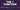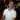# Excel IF Function

April 15, 2017

The IF function is one of the staples of Excel formulas. It’s one of the first formulas people learn when learning how to use Excel. It’s also used EVERYWHERE, and I really mean everywhere. I even wonder if it was one of the first formulas to ever be introduced to Excel so many years ago. At its core, the IF function looks at a condition (evaluated to be True / False) and then takes action if the condition turns out to be true or a different action if the condition results in false.

## Why is this useful?

Many, many, many formulas begin with the IF function. Before the IFERROR function came along, I used to use an IF function to blank out any errors I would receive from my formulas.

``=IF(ISERR(A1),"","My regular formula")``

This is just a simple example, but I would ALWAYS use this when there was no IFERROR function.

There is almost always a reason to use the IF function:

• Are sales low? If so, show “bad”, if they are high, show “good”.
• If the name “Bob” appears in A2, apply a 10% commission on any sales made.
• Is the due date for the current project is within a week? If so, show “INCOMING!”
• You get the idea

The utility of performing one function versus another is fundamental in almost everything we do. “Is it time to go to work? If yes, then leave the house. If not, then read more Spreadsheets Made Easy posts.” When working with our data, clearly we need to make decisions. Decisions are based on “yes / no” criteria. That’s what the IF function helps us do.## How do we use the IF Function?

The IF function is broken up into three main parts:

• The “Yes / No” question asking about your data - this must result in True or False
• The action to take if the “Yes / No” check is “Yes” (i.e. True) - this could be displaying some text, or making a calculation of some kind
• The action to take if the “Yes / No” check if “No” (i.e. False) - again, you can use this to display some text or make a different calculation

### Quick Example

Here we have a simple table that shows employee names, their hourly rate, and how many hours they worked.

We want to calculate any overtime they earned. We can do this is by looking at the hours they worked, then determine if the employee in question worked over 40 hours for the week, and finally apply an overtime rate.

This example uses Excel Tables and Structured References. Check out these posts to learn more:

#### Back to the IF Function

To begin, we’ll start the formula with checking if the Hours Worked are over 40:

``````=IF([@[Hours Worked]]>40
--------------------``````

The underlined portion shows the “Yes / No” question that we’re asking, which is “Are the hours worked over 40?”

Next, we need to tell the formula what to do if the hours worked was indeed over 40:

``````=IF([@[Hours Worked]]>40,([@[Hours Worked]]-40)*[@[Hourly Rate]]*1.5
--------------------  --------------------
Hours over 40         Overtime Rate``````

Now the underlined text shows that we get the hours that were over 40, then multiply by 1.5 times the hourly rate.

What happens if the amount of hours are not over 40? In that case, we want to display 0:

``````=IF([@[Hours Worked]]>40,([@[Hours Worked]]-40)*[@[Hourly Rate]]*1.5,0)
-``````

The last part tells the formula to display 0 if there is no overtime to calculate.

The whole formula with its parts explained is:

``````=IF([@[Hours Worked]]>40, ([@[Hours Worked]]-40)*[@[Hourly Rate]]*1.5, 0)
--------------------  -------------------------------------------  -
The "Yes/No" question                Do this if Yes                 Do this if No``````

And that’s it. If you enjoyed this post, please let me know in the comments below and what you’d like me to work on next.

Wow, you read the whole article! You know, people who make it this far are true learners. And clearly, you value learning. Would you like to learn more about Excel? Please consider supporting me by buying me a coffee (it takes a lot of coffee to write these articles!).Written by Joseph who loves teaching about Excel.